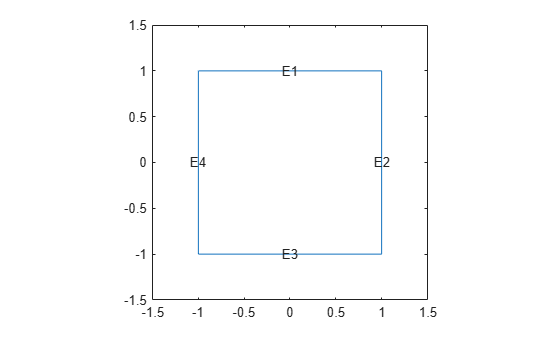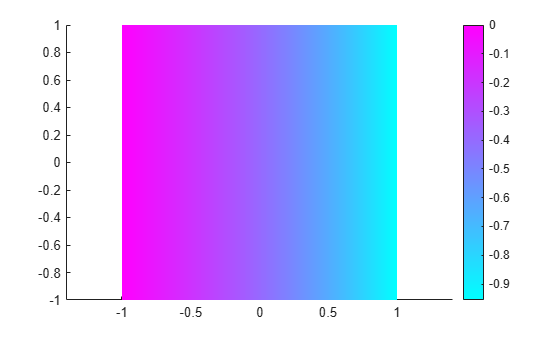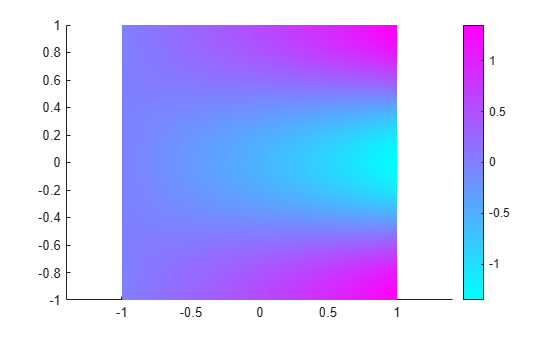Main Content

# Eigenvalues and Eigenmodes of Square

This example shows how to compute the eigenvalues and eigenmodes of a square domain.

The eigenvalue PDE problem is $-\Delta u=\lambda u$. This example finds the eigenvalues smaller than 10 and the corresponding eigenmodes.

Create a model. Import and plot the geometry. The geometry description file for this problem is called `squareg.m`.

```model = createpde(); geometryFromEdges(model,@squareg); pdegplot(model,'EdgeLabels','on') ylim([-1.5,1.5]) axis equal```Specify the Dirichlet boundary condition $u=0$ for the left boundary.

`applyBoundaryCondition(model,'dirichlet','Edge',4,'u',0);`

Specify the zero Neumann boundary condition for the upper and lower boundary.

`applyBoundaryCondition(model,'neumann','Edge',[1,3],'g',0,'q',0);`

Specify the generalized Neumann condition $\frac{\partial u}{\partial n}-\frac{3}{4}u=0$ for the right boundary.

`applyBoundaryCondition(model,'neumann','Edge',2,'g',0,'q',-3/4);`

The eigenvalue PDE coefficients for this problem are c = 1, a = 0, and d = 1. You can enter the eigenvalue range `r` as the vector `[-Inf 10]`.

```specifyCoefficients(model,'m',0,'d',1,'c',1,'a',0,'f',0); r = [-Inf,10];```

Create a mesh and solve the problem.

```generateMesh(model,'Hmax',0.05); results = solvepdeeig(model,r);```
``` Basis= 10, Time= 0.32, New conv eig= 0 Basis= 11, Time= 0.35, New conv eig= 0 Basis= 12, Time= 0.36, New conv eig= 1 Basis= 13, Time= 0.37, New conv eig= 1 Basis= 14, Time= 0.38, New conv eig= 1 Basis= 15, Time= 0.39, New conv eig= 1 Basis= 16, Time= 0.40, New conv eig= 1 Basis= 17, Time= 0.41, New conv eig= 1 Basis= 18, Time= 0.42, New conv eig= 2 Basis= 19, Time= 0.43, New conv eig= 2 Basis= 20, Time= 0.43, New conv eig= 2 Basis= 21, Time= 0.44, New conv eig= 3 Basis= 22, Time= 0.45, New conv eig= 3 Basis= 23, Time= 0.46, New conv eig= 4 Basis= 24, Time= 0.47, New conv eig= 6 End of sweep: Basis= 24, Time= 0.47, New conv eig= 3 Basis= 13, Time= 0.54, New conv eig= 0 Basis= 14, Time= 0.97, New conv eig= 0 Basis= 15, Time= 1.01, New conv eig= 0 Basis= 16, Time= 1.03, New conv eig= 0 Basis= 17, Time= 1.11, New conv eig= 0 Basis= 18, Time= 1.17, New conv eig= 0 Basis= 19, Time= 1.38, New conv eig= 0 Basis= 20, Time= 1.41, New conv eig= 0 Basis= 21, Time= 1.42, New conv eig= 0 Basis= 22, Time= 1.42, New conv eig= 1 Basis= 23, Time= 1.43, New conv eig= 2 End of sweep: Basis= 23, Time= 1.43, New conv eig= 0 Basis= 13, Time= 1.50, New conv eig= 1 Basis= 14, Time= 1.51, New conv eig= 1 Basis= 15, Time= 1.52, New conv eig= 1 Basis= 16, Time= 1.52, New conv eig= 3 Basis= 17, Time= 1.62, New conv eig= 3 Basis= 18, Time= 1.67, New conv eig= 3 Basis= 19, Time= 1.71, New conv eig= 3 Basis= 20, Time= 1.74, New conv eig= 3 Basis= 21, Time= 1.78, New conv eig= 4 End of sweep: Basis= 21, Time= 1.80, New conv eig= 4 Basis= 17, Time= 1.89, New conv eig= 0 End of sweep: Basis= 17, Time= 1.93, New conv eig= 0 ```

There are six eigenvalues smaller than 10 for this problem.

`l = results.Eigenvalues`
```l = 5×1 -0.4146 2.0528 4.8019 7.2693 9.4550 ```

Plot the first and last eigenfunctions in the specified range.

```u = results.Eigenvectors; pdeplot(model,'XYData',u(:,1));````pdeplot(model,'XYData',u(:,length(l)));`This problem is separable, meaning

`$u\left(x,y\right)=f\left(x\right)g\left(y\right).$`

The functions f and g are eigenfunctions in the x and y directions, respectively. In the x direction, the first eigenmode is a slowly increasing exponential function. The higher modes include sinusoids. In the y direction, the first eigenmode is a straight line (constant), the second is half a cosine, the third is a full cosine, the fourth is one and a half full cosines, etc. These eigenmodes in the y direction are associated with the eigenvalues

`$0,\frac{{\pi }^{2}}{4},\frac{4{\pi }^{2}}{4},\frac{9{\pi }^{2}}{4},...$`

It is possible to trace the preceding eigenvalues in the eigenvalues of the solution. Looking at a plot of the first eigenmode, you can see that it is made up of the first eigenmodes in the x and y directions. The second eigenmode is made up of the first eigenmode in the x direction and the second eigenmode in the y direction.

Look at the difference between the first and the second eigenvalue compared to ${\pi }^{2}/4$:

`l(2) - l(1) - pi^2/4`
```ans = 1.6751e-07 ```

Likewise, the fifth eigenmode is made up of the first eigenmode in the x direction and the third eigenmode in the y direction. As expected, `l(5)-l(1)` is approximately equal to ${\pi }^{2}$:

`l(5) - l(1) - pi^2`
```ans = 6.2135e-06 ```

You can explore higher modes by increasing the search range to include eigenvalues greater than 10.

## SupportGet trial now